Students can download Maths Chapter 1 Fractions Ex 1.2 Questions and Answers, Notes, Samacheer Kalvi 6th Maths Guide Pdf helps you to revise the complete Tamilnadu State Board New Syllabus, helps students complete homework assignments and to score high marks in board exams.

## Tamilnadu Samacheer Kalvi 6th Maths Solutions Term 3 Chapter 1 Fractions Ex 1.2

Miscellaneous Practice Problems

Question 1.
Sankari purchased 2$$\frac{1}{2}$$ m cloth to stich a long skirt and 1$$\frac{3}{4}$$ m cloth to stitch blouse. If the cost is Rs. 120 per metre then find the cost of cloth purchased by her.
Solution:
Total cloth purchased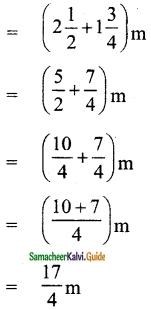cost of 1 metre = Rs. 120
Total cost of cloth purchased
= Rs. 120 × $$\frac{17}{4}$$
= Rs. 510Question 2.
From his office, a person wants to reach his house on foot which is at a distance of 5$$\frac{3}{4}$$ km. If he had walked 2$$\frac{1}{2}$$ km, how much distance still he has to walk to reach his house?
Solution: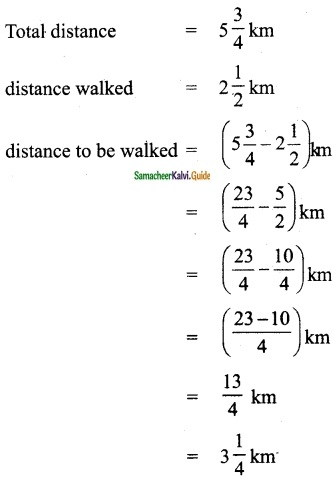Question 3.
Which is smaller? The difference between 2$$\frac{1}{2}$$ and 3$$\frac{2}{3}$$ or the sum of 1$$\frac{1}{2}$$ and 2$$\frac{1}{4}$$.
Solution: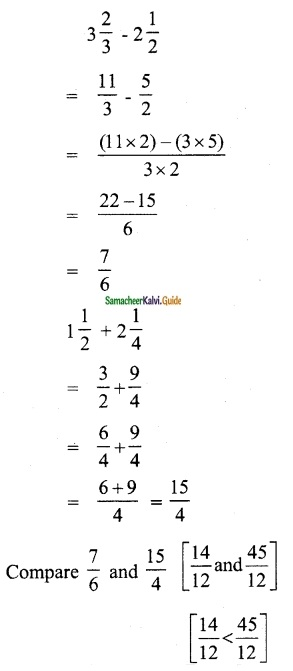∴ The difference of 2$$\frac{1}{2}$$ and 3$$\frac{2}{3}$$ is smallerQuestion 4.
Mangai bought 6$$\frac{3}{4}$$ kg of apples. If Kalai 1 bought 1$$\frac{1}{2}$$ times a Mangai bought, then how many kilograms of apples did Kalai buy?
Solution:
Apples bought by Mangai = 6$$\frac{3}{4}$$ kg
Apples bought by Kalai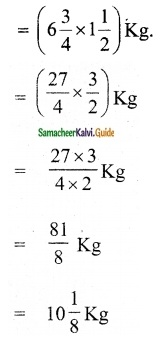Question 5.
The length of the staircase is 5$$\frac{1}{2}$$ m. If one step is set at $$\frac{1}{4}$$ m, then how many steps will be there in the staircase?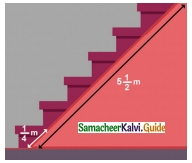Solution:
Total length of the staircase = 5$$\frac{1}{2}$$ m
length of each step = $$\frac{1}{4}$$ m
No of steps in the stair case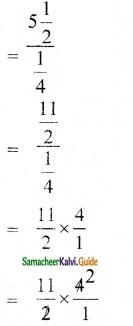= 22 stepsChallenge Problems

Question 6.
By using the following clues, find who am I?
(i) Each of my numerator and denominator is a single-digit number.
(ii) The sum of my numerator and denominator is a multiple of 3.
(iii) The product of my numerator and denominator is a multiple of 4.
Solution:
The numerator may be any one of!, 2, 3,4, 5, 6, 7, 8, 9 and the denominator may be any one of 1, 2,3,4, 5,6, 7,8,9. Sum of numerator and denominator is a multiple of 3.
∴ Possible proper fractions are $$\frac{1}{2}, \frac{1}{5}, \frac{1}{8}, \frac{2}{4}, \frac{2}{7}, \frac{3}{6}, \frac{3}{9}, \frac{4}{5}, \frac{4}{8}, \frac{5}{7}, \frac{6}{9}$$
Also given the product of numerator and denominator is a multiple of 4.
∴ Possible fractions are $$\frac{1}{8}, \frac{2}{4}, \frac{4}{5}, \frac{4}{8}$$

Question 7.
Add the difference between 1$$\frac{1}{3}$$ and 3$$\frac{1}{6}$$ and the difference between 4$$\frac{1}{6}$$ and 2$$\frac{1}{3}$$
Solution: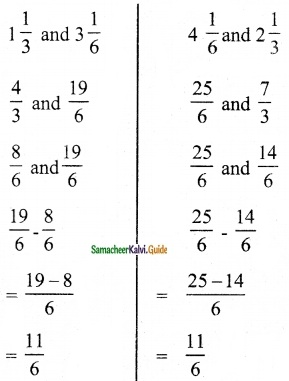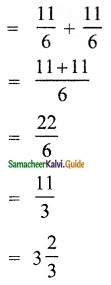Question 8.
What fraction is to be subtracted from 9$$\frac{3}{7}$$ to get 3$$\frac{1}{5}$$?
Solution:
Let the fraction be x
According to the problem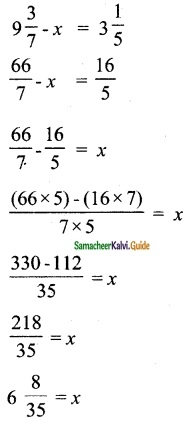The fraction to be subtracted is 6$$\frac{8}{35}$$Question 9.
The sum of two fractions is 5$$\frac{3}{9}$$. If one of the fractions is 2$$\frac{3}{4}$$, find the other fraction.
Solution:
Let the other fraction be x
According to the problem,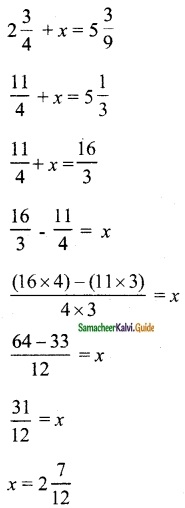∴ The other fraction is 2$$\frac{7}{12}$$Question 10.
By what number should 3$$\frac{1}{16}$$ be multiplied to get 9$$\frac{3}{16}$$?
Solution:
Let the number be x
According to the problem,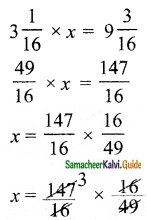x = 3
The number is 3

Question 11.
Complete the fifth row in the Leibnitz triangle which is based on subtraction.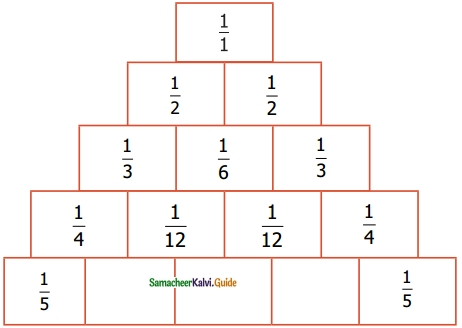Solution: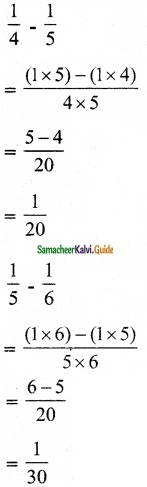Question 12.
A painter painted $$\frac{3}{8}$$ of the wall of which one third is painted in yellow colour. What fraction is the yellow colour of the entire wall?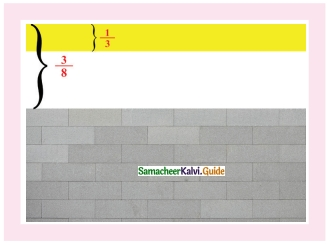Solution:
yellow colour of the entire wall
= $$\frac{3}{8}$$ × $$\frac{1}{3}$$
= $$\frac{1}{8}$$

Question 13.
A rabbit has to cover 26$$\frac{1}{4}$$ m to fetch its food. If it covers 1$$\frac{3}{4}$$ m in one jump, then how many jumps will it take to fetch its food?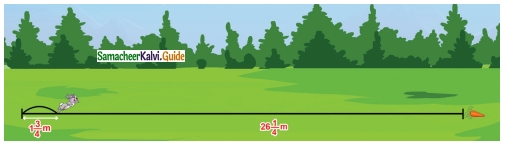Solution:
Total distance = 26$$\frac{1}{4}$$ m
Distance covered in one jump = 1$$\frac{3}{4}$$ m
Number of jumps required to fetch the food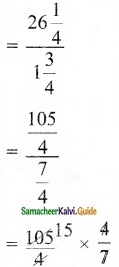= 15Question 14.
Look at the picture and answer the following questions.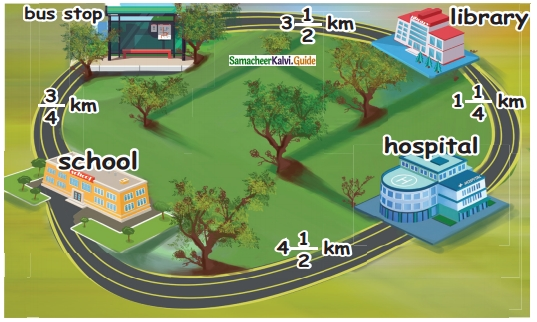(i) What is the distance from the school to Library via Bus stop?
(ii) What is the distance between School and Library via Hospital?
(iii) Which is the shortest distance between (i) and (ii)?
(iv) The distance between School and Hospital is times the distance between School and Bus stop.
Solution: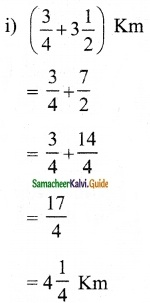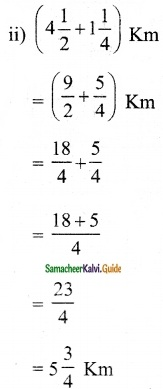(iii) Via bus stop
(iv) 6 times (6 × $$\frac{3}{4}$$ = $$\frac{18}{4}$$ = $$\frac{9}{2}$$ = 4$$\frac{1}{2}$$)